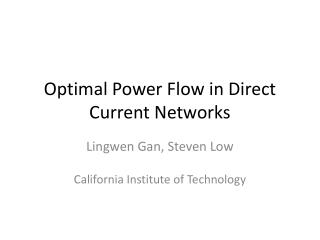DownloadDownload PresentationOptimal Power Flow in Direct Current Networks

# Optimal Power Flow in Direct Current Networks

Télécharger la présentation## Optimal Power Flow in Direct Current Networks

- - - - - - - - - - - - - - - - - - - - - - - - - - - E N D - - - - - - - - - - - - - - - - - - - - - - - - - - -
##### Presentation Transcript

1. Optimal Power Flow in Direct Current Networks Lingwen Gan, Steven Low California Institute of Technology

2. Optimal power flow (OPF) What is OPF? Schedule power plants, minimize electricity cost. Is OPF important? Solved every 15 minutes, underlies power system operation.

3. Challenges in solving OPF Power flow governed by nonlinear (nonconvex) laws Three ways to deal with the challenge 1. Linearization / Approximation. [DC, …] 2. Local optima [Newton-Raphson, SQP, …] May guarantee global optimality 3. Convex relaxation [SDP, SOCP]

4. Outline Formulate OPF and convex relaxations. Sufficient condition for exact convex relaxations. Modify OPF accordingly.

5. Terminologies in power network Power network represented by a graph. bus line j i Direct current: V, s, y are real.

6. The OPF problem min generation cost s V over power flow constraints s.t. j i power injection constraints Nonconvex! voltage constraints

7. Semi-definite programming(SDP) relaxation Feasible set of OPF OPF SDP [Lavaei10] min Feasible set of SDP min s.t. s.t. …… …… …… remove rank 1

8. Second-order cone programming(SOCP) relaxation Feasible set of SDP optimal solution Feasible set of SOCP Def: SOCP exact (every) optimal solution feasible for OPF.

9. Literature review Counter examples exist. Sufficient conditions derived. Theorem [Lavaei10]:  SDP exact. ×

10. A sufficient condition for exact SOCP Affine in s. Theorem: at SOCP solutions  SOCP exact. Proof: SOCP not exact  exists SOCP solution (s,W) infeasible for OPF.  construct (s’,W’) with Obj(s’,W’) < Obj(s,W). cannot be checked a priori.

11. A modified OPF Impose as constraints! OPF-m Theorem: SOCP is alwaysexact for OPF-m.

12. Summary Propose an SOCP relaxation for OPF in direct currentnetworks. Provide a sufficient condition for exact SOCP. Propose a modified OPF whose SOCP is always exact. Thank you!